# CHAPTER 9 - Introduction to Rational Index

In the previous section, we discussed the expansion of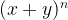${(x + y)^n}$, where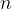$n$ is a natural number. We’ll extend that discussion to a more general scenario now. In particular, we’ll consider the expansion of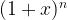${(1 + x)^n}$, where$n$ is a rational number and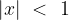$|x|\,\, < \,\,1$. Note that any binomial of the form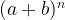${(a + b)^n}$ can be reduced to this form.: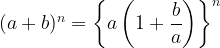${(a + b)^n} = {\left\{ {a\left( {1 + \dfrac{b}{a}} \right)} \right\}^n}$ (we are assuming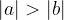$|a| > |b|$)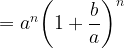$= {a^n}{\left( {1 + \dfrac{b}{a}} \right)^n}$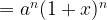$= {a^n}{(1 + x)^n}$ where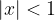$|x|<1$
The general binomial theorem states that$\begin{array}{l} {(1 + x)^n} = 1 + nx + \dfrac{{n(n - 1)}}{{2!}}{x^2} + \dfrac{{n(n - 1)(n - 2)}}{{3!}}\;{x^3} + \ldots \;\\ \\ \;\;\;\;\;\;\;\;\;\;\;\;\;\;\;\;\ldots + \dfrac{{n(n - 1)(n - 2)\ldots (n - r + 1)}}{{r!}}{x^r} + \ldots \infty \end{array}$
That is, there are an infinite number of terms in the expansion with the general term given by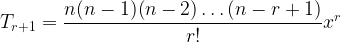${T_{r + 1}} = \dfrac{{n(n - 1)(n - 2)\ldots (n - r + 1)}}{{r!}}{x^r}$
For an approximate proof of this expansion, we proceed as follows: assuming that the expansion contains an infinite number of terms, we have: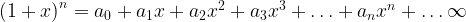${\left( {1 + x} \right)^n} = {a_0} + {a_1}x + {a_2}{x^2} + {a_3}{x^3} +\ldots + {a_n}{x^n} +\ldots \infty$
Putting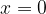$x = 0$ gives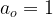${a_o} = 1$. Now differentiating once gives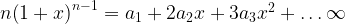$n{\left( {1 + x} \right)^{n - 1}} = {a_1} + 2{a_2}x + 3{a_3}{x^2} + \ldots \infty$
Putting$x = 0$ gives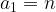${a_1} = n$.
Proceeding in this way, we find that the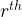${r^{th}}$ coefficient is given by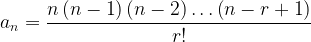${a_n} = \dfrac{{n\left( {n - 1} \right)\left( {n - 2} \right)\ldots \left( {n - r + 1} \right)}}{{r!}}$
Note that if$n$ is a natural number, then this expansion reduces to the expansion obtained earlier, because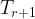${T_{r + 1}}$ becomes$^n{C_r}{x^r}$, and the expansion terminates for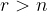$r > n$. For the general${T_{r + 1}}$, we obviously cannot use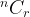$^n{C_r}$ since that is defined only for natural$n$.
One very important point that we are emphasizing again is that the general expansion holds only for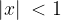$|x|\; < 1$.
Let us denote the genral binomial coefficient by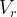${V_r}$. Thus, we have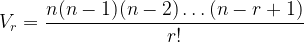${V_r} = \dfrac{{n(n - 1)(n - 2) \ldots (n - r + 1)}}{{r!}}$ and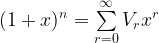${(1 + x)^n} = \sum\limits_{r = 0}^\infty {} {V_r}{x^r}$
Let us discuss some particularly interesting expansions. In all cases,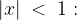$|x|\; < \;1:$
(1)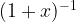${(1 + x)^{ - 1}}$
Since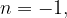$n = - 1,$ we see that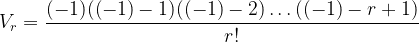${V_r} = \dfrac{{( - 1)(( - 1) - 1)(( - 1) - 2) \ldots (( - 1) - r + 1)}}{{r!}}$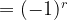$= {( - 1)^r}$
so that the expansion is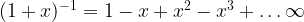${(1 + x)^{ - 1}} = 1 - x + {x^2} - {x^3} + \ldots \infty$
(2)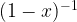${(1 - x)^{ - 1}}$
Again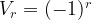${V_r} = {( - 1)^r}$ and thus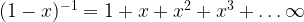${(1 - x)^{ - 1}} = 1 + x + {x^2} + {x^3} +\ldots \infty$
(3)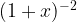${(1 + x)^{ - 2}}$
We have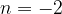$n = - 2$;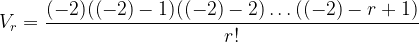${V_r} = \dfrac{{( - 2)(( - 2) - 1)(( - 2) - 2) \ldots (( - 2) - r + 1)}}{{r!}}$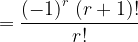$= \dfrac{{{{( - 1)}^r}\;(r + 1)!}}{{r!}}$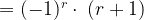$= {( - 1)^r} \cdot \;(r + 1)$
Thus,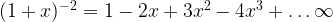${(1 + x)^{ - 2}} = 1 - 2x + 3{x^2} - 4{x^3} + \ldots \infty$
(4)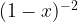${(1 - x)^{ - 2}}$
Again${V_r} = {( - 1)^r} \cdot (r + 1)$ so that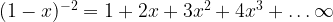${(1 - x)^{ - 2}} = 1 + 2x + 3{x^2} + 4{x^3} +\ldots \infty$

### Related Posts

There is no other posts in this category.